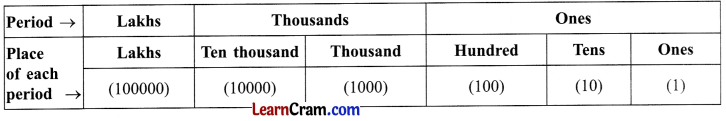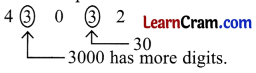# DAV Class 4 Maths Chapter 1 Brain Teasers Solutions

The DAV Class 4 Maths Book Solutions and DAV Class 4 Maths Chapter 1 Brain Teasers Solutions of Numbers up to 999999 offer comprehensive answers to textbook questions.

## DAV Class 4 Maths Ch 1 Brain Teasers Solutions

Question 1.
(a) The greatest 6-digit number formed by using the digits 7, 3, 1, 0, 9 and 4 is:
(i) 974301
(ii) 974310
(iii) 974103
(iv) 973410
(ii) 974310

(b) The period of the digit ________ in 6,54,321 is Lakhs.
(i) 5
(ii) 3
(iii) 4
(iv) 6
(iv) 6

(c) The smallest 6-digit number is_______
(i) 1,11,111
(ii) 1,00,001
(iii) 1,10,010
(iv) 1,00,000
(iv) 1,00,000

(d) The sum of the place value of 9 and 7 in the number 947635 is ________
(i) 970000
(ii) 907000
(iii) 101000
(iv) 900700
(ii) 907000

(e) 8 × 1,00,000 + 5 × 10,000 + 6 × 1,000 + 9 × 100 + 0 + 5 is equal to ________
(i) 856095
(ii) 856905
(iii) 850695
(iv) 865905
(ii) 856905Question 2.
Write the three periods of a 6-digit numeral. Also mention the corresponding places of each period
3 periods of a 6-digit numeral areQuestion 3.
You are given the following numerals. Pick out the greatest numeral and the smallest numeral from the set.Greatest numeral – 99,950
Smallest numeral – 509

Question 4.
Fill in the blanks.
(a) The smallest 5-digit numeral is ________
10,000

(b) (i) 1 lakh = ________ thousands
(ii) ________ hundred = 10 tens
(i) 100
(ii) 1

(c) The numeral just before 90,000 is ________
89,999

(d) The places, thousands and ten thousands belong to the ________
thousand

(e) Compare by using “<” or “=” in the box.
(i) 11,111 ________ 1,111
(ii) 9,87,091 ________ 9, 78,091
(i) >
(ii) >

Question 5.
Write down the numeral which is one more than the greatest 5-digit numeral.
Greatest 5-digit numeral = 99999
Numeral one more than this numeral
= 99999 + 1
= 1,00,000

Question 6.
Which numeral has more digits greatest 5-digit numeral or smallest 6-digit numeral?
Smallest 6-digit numeral i.e. 1,00,000.Question 7.
Write the standard numeral for:
(a) 5,000 + 20 + 3
(b) 600 + 70 + 15
(c) 70,000 + 7 + 700 + 70
(a) 5,023;
(b) 685;
(c) 70,777

Question 8.
Write down the period and place of the underlined digit in each numeral.

 Numeral Period Place (a) 92,958 0b) 87,215 (c) 63,049 (d) 1,00,000 (e) 53,269

 Numeral Period Place (a) 92,958 Ones Hundreds 0b) 87,215 Ones Tens (c) 63,049 Thousands Ten thousands (d) 1,00,000 Lakh Lakh (e) 53,269 Ones Ones

Question 9.
Compare the place value of the encircled digits in the numeral 43o32.
For comparison we first count the digits.∴ 3000 > 30
3 thousand > 3 tens

Question 10.
You are given some digits below. Form the greatest and smallest 5-digit numeral using each one of these digits only once.Greatest 5-digit numeral = 95,430
Smallest 5-digit numeral = 30,459

Question 1.
Write the following in expanded form and write the place value of 2 in each case.
(a) 5,20,673
(b) 7,08,320
(c) 2,40,088
(a) 5,20,673 = 5,00,000 + 20,000 + 0 + 600 + 70 + 3
Place value of 2 = 20,000

(b) 7,08,320 = 7,00,000 + 0 + 8,000 + 300 + 20 + 0
Place value of 2 = 20

(c) 2,40,088 = 2,00,000 + 40,000 + 0 + 0 + 80 + 8
Place value of 2 = 2,00,000Question 2.
Take the digits 5, 6, 0 and 4. Make any 2 numbers each with 8 digits.
8-digit numbers are 56644005, 66554400

Question 3.
Write in short form:
(a) 5,00,000 + 0 + 3,000 + 200 + 70 + 1
(b) 7,00,000 + 30,000 + 8,000 + 0 + 40 + 3
(c) 3,00,000 + 50,000 + 0 + 0 + 20 + 0
(d) 6,00,000 + 90,000 + 7,000 + 0 + 0 + 4
(a) 503271
(b) 738043
(c) 350020
(d) 697004

Question 4.
Write the numeral for the following number names:
(a) Two lakh nine thousand eighteen.
(b) Five lakh twenty three thousand eight hundred six.
(c) Three lakh forty five thousand and six.
(d) Eight lakh nine thousand fifty eight.
(a) 2,09,018
(b) 5,23,806
(c) 3,45,006
(d) 8,09,058

Question 5.
Arrange the following numbers in ascending order:
(a) 98,002; 2,10,999; 98,073; 98,103.
(b) 2,20,222; 2,22,122; 2,12,222; 2,22,102.